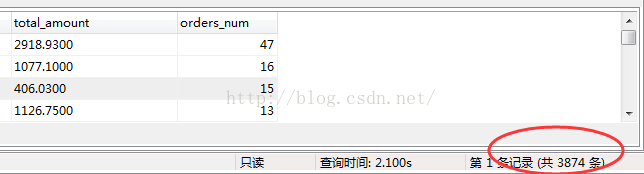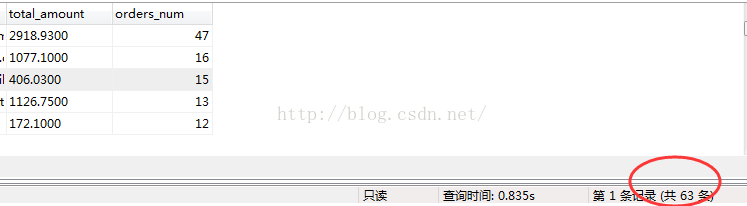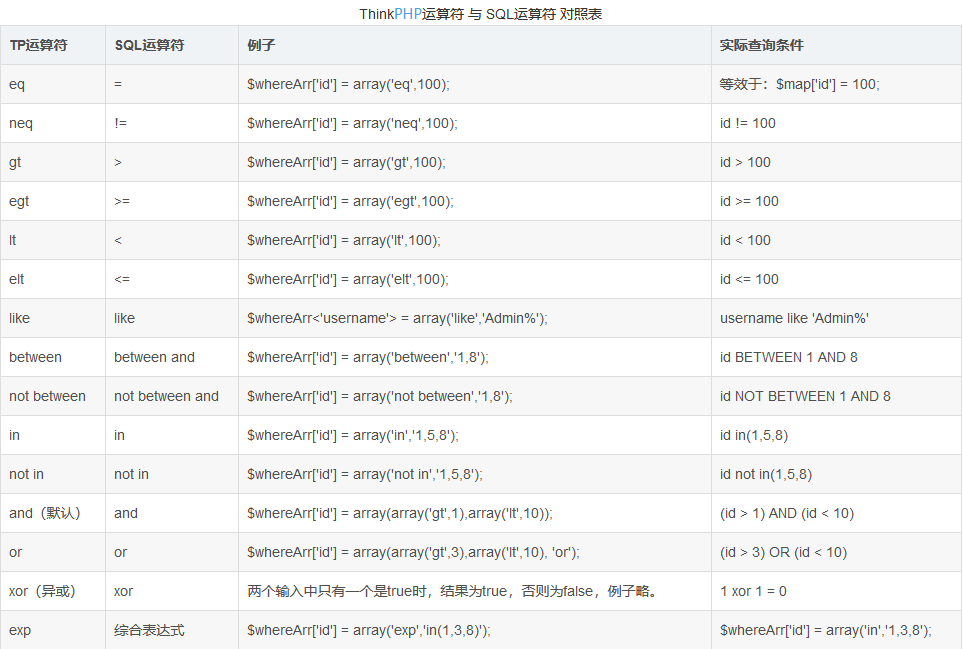• where怎么用
千次阅读
2017-03-17 11:08:37

SELECT *
FROM tbllog_player
WHERE find_in_set( 3 , (select group_concat(role_id) from tbllog_player))MySQL where
更多相关内容
• select * from (select (select t.gc_name from shop_goods_class as t where t.gc_id=(select two_gc.gc_parent_id from shop_goods_class as two_gc where two_gc.gc_id=gc.gc_parent_id) ) as oneName, ...

参考  http://blog.csdn.net/github_26672553/article/details/51889847

SELECT customer_id,group_id,email,SUM(grand_total) AS total_amount,COUNT(*) AS orders_num
FROM sales_flat_order_grid
LEFT JOIN customer_entity  ON customer_entity.entity_id = sales_flat_order_grid.customer_id
WHERE status='complete' AND customer_id IS NOT NULL  AND customer_entity.group_id = 1
GROUP BY customer_id
ORDER BY orders_num DESC需求：

我只需要orders_num这列值 >= 5的数据。

方法：在这个select外面 在套一个 select

SELECT SS.* FROM (
#...上面那个SQL
)SS
where SS.orders_num >=5   
最终如下：
SELECT SS.* FROM (
SELECT customer_id,group_id,email,SUM(grand_total) AS total_amount,COUNT(*) AS orders_num
FROM sales_flat_order_grid
LEFT JOIN customer_entity  ON customer_entity.entity_id = sales_flat_order_grid.customer_id
WHERE status='complete' AND customer_id IS NOT NULL  AND customer_entity.group_id = 1
GROUP BY customer_id
ORDER BY orders_num DESC
)SS
where SS.orders_num >=5优化前：

select  (select t.gc_name from shop_goods_class as t where t.gc_id=(select two_gc.gc_parent_id from shop_goods_class as two_gc where two_gc.gc_id=gc.gc_parent_id) ) as oneName,

(select two_gc.gc_name from shop_goods_class as two_gc where two_gc.gc_id=gc.gc_parent_id) as twoGcName,
(select two_gc.gc_id from shop_goods_class as two_gc where two_gc.gc_id=gc.gc_parent_id) as id,
(select two_gc.gc_parent_id from shop_goods_class as two_gc where two_gc.gc_id=gc.gc_parent_id) as pid,
gc.gc_name as threeGcName from shop_goods_class as gc where   (select two_gc.gc_name from shop_goods_class as two_gc where two_gc.gc_id=gc.gc_parent_id) is not null

优化后的sql：
select * from (select  (select t.gc_name from shop_goods_class as t where t.gc_id=(select two_gc.gc_parent_id from shop_goods_class as two_gc where two_gc.gc_id=gc.gc_parent_id) ) as oneName,
(select two_gc.gc_name from shop_goods_class as two_gc where two_gc.gc_id=gc.gc_parent_id) as twoGcName,
gc.gc_name as threeGcName from shop_goods_class as gc )tt
where tt.twoGcName is not null  and tt.oneName is not null

展开全文• OR查询 $where['userid|uname'] = ['like',"%".$keyword."%"]; $list = Db::name('member')->where($where)->order('mid desc')->limit(15)->select();$where['userid|uname'] = ['like',"%".$keyword."%"];

$list = Db::name('member')->where($where)->order('mid desc')->limit(15)->select(); 
$where['field'] = ['neq','not null']; //不为空$where['field'] = null;       //null

$where = array();$where['id'] = array('in', $uid_str);$res = $this->db2->name('user')->where($where)->select();

展开全文php
• # coding:utf-8 import numpy as np x = np.array([[1, -1], [-1, 1]]) print(x) """ [[ 1 -1] [-1 1]] """ ...x[:, 0] = np.where(x[:, 0] == -1, 100, x[:, 0]) print(x) """ [[ 1 -1] [10...
# coding:utf-8

import numpy as np

x = np.array([[1, -1], [-1, 1]])
print(x)
"""
[[ 1 -1]
[-1  1]]
"""

# 将第一列中的-1替换程100
x[:, 0] = np.where(x[:, 0] == -1, 100, x[:, 0])
print(x)
"""
[[  1  -1]
[100   1]]
"""

# 将第一行中的-1替换成200
x[0, :] = np.where(x[0, :] == -1, 200, x[0, :])
print(x)
"""
[[  1 200]
[100   1]]
"""

# 将除去100和200之外的所有元素替换成300
x = np.where((x != 100) & (x != 200), 300, x)
print(x)
"""
[[300 200]
[100 300]]
"""

# 将100和200替换成400
x = np.where((x == 100) | (x == 200), 400, x)
print(x)
"""
[[300 400]
[400 300]]
"""

参考链接

展开全文python
• ## ABAP 动态where使用

千次阅读 2019-09-22 09:46:42
REPORT ztest001_xch. TABLES: makt. ...DATA where_tab(80) OCCURS 10 WITH HEADER LINE. DATA : wa_itab LIKE makt OCCURS 10 WITH HEADER LINE. PARAMETERS: s_trx LIKE makt-matnr OBLIGATORY, la...
• ## mapper where使用

千次阅读 2018-12-11 09:57:54
&lt;where&gt; &lt;if test= "id!=null"&gt; AND t1.fid = #{id,jdbcType=VARCHAR} &lt;/if&gt; &lt;/where&gt; jdbctype 一定要写
• ## where后面使用别名

千次阅读 2020-12-13 17:06:40
Oracle中SQL语句使用别名是是一件十分常见的事。如： SQL> select ename,sal as salary from emp where sal<1000; ENAME          SALARY ---------- -----...
• 注意： df1.where(cond,df2) 等价于 np.where(cond, df1, df2) 1. pandas.DataFrame.where DataFrame.where(cond, other=nan, inplace=False, axis=None, level=None, try_cast=False, raise_on_error=True) 功能...
• ## Oracle where if

千次阅读 2021-10-27 09:07:37
Oracle where不能如其他sql直接添加if逻辑,只能使用case when select * from table_data t where (case when t.column_data='条件' then t.column_data when t.column_data='条件2' then t.rep_data )='条件3' ...oracle
• I am trying to SELECT the the rows where a certain condition is met multiple times and how many times that condition is met.For example, for the table:Animal ID NumKids PostCodeCow 12...
• 同BETWEEN关键字一样，IN的引入也是为了更方便地限制检索数据的范围，灵活使用IN关键字，可以简洁的语句实现结构复杂的查询。 语法格式为： 表达式 [NOT] IN (表达式1 , 表达式2 [,…表达式n])【例】查询所有...
• SELECT * FROM A WHERE ( CASE WHEN PSTYP = '9' THEN POSID1 WHEN PSTYP = '0' THEN POSID2 END ) = '1' DECODE(列名, 值1, 返回值1,值2,返回值2,默认值)
• 在Laravel中的可以同时使用多个where,所以我们可以每个字段分配一个where() 然后在每个where()中去闭包判断 $username = '';// 收货人姓名$hospital_id = ''; // 医院id # 判断是否有姓名搜索 if (!empty(\$request...laravel php
• 您可以使用CASE如下：WHERE OrderNumber LIKE CASE WHEN IsNumeric(@OrderNumber) = 1 THEN @OrderNumber ELSE '%' + @OrderNumber END或者你可以使用像@JReed指出的IF语句。你应该可以做到这一点，没有任何IF或...
• 2. WHERE可以单独作为过滤条件进行使用； 单独使用不会报错； Having单独使用报错很明显，需要GROUP BY语句联合一起使用；并不能单独使用； 3. HAVING它弥补了WHERE关键字不能与聚合函数联...Oracle where Having
• select currentlyvalue from fw_sysconfig where PARAMCODE=‘isDegreeCourse’ –注：currentlyvalue=‘是’ 下面sql中使用条件：isdegreecourse = ‘是’ ；否则使用条件 studytypecode = 1 SELECT * FROM base_...
• 当我们使用某个表达式作为输出的一列时,我们无法再Where条件中直接使用该列作判断条件. 例如下面的SQL语句: select id, (c1 + c2) as s from t1 where s > 100 SQL Server 报错: “列名 s 无效” 当然,写成 ...where 别名
• 先来了解Mysql and与or的使用 AND和OR可在WHERE子语句中把两个或多个条件结合起来 使用OR关键字时： 只要符合这几个查询条件的其中一个条件，这样的记录就会被查询出来。 如果不符合这些查询条件的任何一条，这样的...php mysql thinkphp
• select spj.jno from spj where spj.sno in( select sno from spj where spj.pno in( select pno from p where color='red') 二、查询至少使用了厂家S1所提供的全部零件的工程名 not exists 与双重否定的使用 ...oracle
• 文章目录背景介绍问题解决方式一：将where中的别名换成表达式即可解决方式二：使用子查询的方式，然后就可以在where字句中调用了问题拓展总结 背景介绍 昨天在写sql脚本，与前端进行调试的时候发现，SQL中的字段别名...SQL执行顺序 mysql字段别名
• ## WHERE子查询

千次阅读 2019-03-07 15:21:09
WHERE子查询： 查询出工资低于平均工资的雇员信息： SELECT * FROM emp WHERE sal&lt;( SELECT AVG(sal) FROM emp); 查询出公司最早入职的雇员信息： SELECT * FROM emp WHERE hiredate=( SELECT MIN...
• mysql 在select 和 where条件中如何使用 IF 条件语句
• where字句常用的查询条件 查询条件 谓词 比较 = , > , < , >= , <= , （<> , !=不等于） 确定范围 BETWEEN AND(相当于闭集合[BETWEEN,AND]) , NOT BETWEEN AND(不属于闭集合[BETWEEN,...mysql where
• MySQL中WHERE子句条件判断<>，!= 二者的区别 ANSI标准中是<>(所以建议<>)，但为了跟大部分数据库保持一致，数据库中一般都提供了 !=(高级语言一般用来表示不等于) 与 <> 来表示不等于：...
• mysqldump 的 where 条件子句适用于这种情况：导出某张表的部分数据。...where 条件的使用和 sql 语句中 where 是相同的。 语法如下： 1.mysqldump -u username -p password -B dbname --tables tbl --wh
• 有时候我们对查询的结果进行了处理形成了新的列名，又想在查询条件中使用此列别名，但MySQL是不支持这样做的。 为什么不支持呢？之所以MySQL中不允许使用列别名作为查询条件，据说是因为MySql中列的别名本来是返回...mysql 别名
• ## numpy之where的用法

千次阅读 2020-07-04 12:50:37
1、numpy.where的返回结果 numpy.where调用方式为numpy.where(condition,1,2) 满足条件的位置上返回结果1，不满足的位置上返回结果2  例如通过where()函数将a数组...zip函数直接返回的是一个对象，可以过for循环python
• ## 不要再用where 1=1了，有更好的写法

千次阅读 热门讨论 2022-03-01 07:55:39
刚入行的同学，看到在SQL语句中出现where 1 = 1这样的条件可能会有所困惑，而长时间这样使用的朋友可能又习以为常。 那么，你是否还记得当初为什么要这样写？是否有性能问题？有没有更好的写法？ 今天这篇文章，带...MySQL mysql优化
• 本文教你使用Linux操作系统中的Whereis命令，包括注意事项。whereis是一个命令行实用程序，允许你查找给定命令的二进制文件、源文件和手动页面文件的位置。如何使用whereis命令及注意事项whereis命令的语法如下：......